Next: 4. On-Line Help Up: 1. Introductory Material Previous: 2. Distribution

Subsections

# 3. A Brief Tutorial

In this tutorial, we will assume that you have the X Window System running on your workstation. If your workstation can run X but it is not installed, enlist the aid of a system guru to get him or her to set things up for you. Although it is possible to run IMPS from a dumb terminal (for example, to work from home, where mere mortals only have terminal emulators), you will miss the functionality that X provides, such as fancy TEX previewing of formulas and proofs, and interaction with IMPS using the mouse and the menu facility. We will also assume that you have the shell variable IMPS correctly set.

To start IMPS enter this command at the shell prompt:

$IMPS/../bin/start_imps Executing this command will do the following: • Start an Emacs session. • Start IMPS (which is actually a Tea'' process) subordinate to Emacs. • Make two new directories in your home directory:$HOME/imps and $HOME/imps/theories needed for the IMPS exercises. • Add some files to your /tmp directory. • Start one or more iconified TEX preview windows. To quit IMPS type C-x C-c. This will kill Tea and the Emacs process. If you are trying to learn to use IMPS, it would be a good idea to work through the exercises described in Chaptersand 5, after reading this tutorial. # 3.1 Interacting with IMPS Most of your interaction with IMPS can be initiated by selecting of one of a number of menu options. The menu format depends on the version of Emacs you are using. • Free Software Foundation version 193.1 menus are invoked by clicking on the menubar. The resulting menu has a number of options. • Version 18 menus are invoked by clicking the right mouse button on the mode line. Moreover, the menu consists of one or more panes which are stacked like cards; each individual pane has a label plus a number of options. • Lucid version 19 menus are invoked by clicking on the menubar. The resulting menu has a number of options. Some of the options open up to new submenus. These three menu systems are quite similar to each other; it is easy to translate instructions for one menu system into instructions for the other. You select an option within a menu or pane by pointing with the mouse (notice the selected option is enclosed in a box) and clicking the right mouse button. If you do not want to select anything after the menu appears, click right on the option CANCEL MENU REQUEST (or just click right when the mouse points to somewhere off the menu.) This will cause the menu to disappear. Notice that some of the options are followed by a sequence of keystrokes enclosed in parentheses. This means that these options can also be invoked directly using these keystrokes. As you become more familiar with IMPS, you will probably want to use these more direct invocations. Note that you can use the menus to change buffers or modify the number of windows on the screen. You should also be aware that the panes which appear on the menu, and the options within each pane, usually depend on the window you clicked on to bring up the menu. # 3.2 On-line Documentation The following documentation is available on-line: • The User's Manual. If you have a TEX previewer, the IMPS manual 3.2 is available on-line. You can locate specific items in the manual by clicking right on the option IMPS manual entry in the pane Help. • Def-forms. A def-form is essentially a specification of an IMPS entity. In the course of your interaction with IMPS you may want to examine the definition of some object. You can locate the def-form which specifies it by clicking right on the option Find def-form (C- .) in the pane Help. For more details on on-line documentation, see Chapter 4. # 3.3 Languages and Theories For now, you can think of a theory as a mathematical object consisting of a language and a set of closed formulas in that language called axioms. For example, one of the last things that was printed out in the startup procedure was the line  Current theory: #{imps-theory 3: H-O-REAL-ARITHMETIC}  h-o-real-arithmetic is a basic IMPS theory which contains an axiomatic theory of the real numbers, characterized as a complete ordered field with the integers and rationals as imbedded structures. This is a fairly extensive theory, so we only describe it informally here. The atomic sorts of the language are N, Z, Q, R denoting the natural numbers, integers, rationals, and reals. The language constants of this theory are of three kinds: • The primitive function and relation constants +,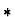, /,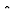,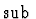, -, <,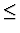that denote the arithmetic operations of addition, multiplication, division, exponentiation, subtraction, negation, and the binary predicates less than, and less than or equal to, respectively. • An infinite set of individual constants, one for each rational number. • Various defined constants such as the operator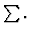The domains of the primitive constants and some of the defined constants are given in Table 3.1. Table 3.1: Domains of Arithmetic Operators.  Operator Domain +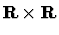star-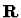sub^ E /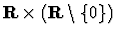sum S prod S ! N <<=>>=where: •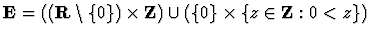•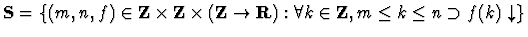The axioms of this theory are the usual field and order axioms as well as the completeness axiom which states that any predicate which is nonvacuous and bounded above has a least upper bound. This theory also contains the full second-order induction principle for the integers as an axiom. At any given time there is a theory that IMPS regards as the current theory. For example, as we mentioned above, the current theory is h-o-real-arithmetic when IMPS finishes loading. The current theory affects the behavior of IMPS in two very significant ways: • Any expression that is read in by IMPS belongs to the language of the current theory (unless another language is explicitly specified). • In any proof that you begin, you may avail yourself of all the axioms of the current theory and all the theorems previously proved and entered in the current theory. You can change the current theory by selecting the Set current theory option of the pane General. When you are finished playing around with changing theories, set the current theory back to h-o-real-arithmetic, which is needed for the tutorial. # 3.4 Syntax Mathematicians and logicians usually think of a formula as a syntactic object represented by some text such as x+y=y+x. For IMPS, on the other hand, a formula is a complex data structure, essentially a record, with numerous fields. To build a formula in a convenient way, you need to have a parser. A parser takes a formula represented as a string and produces a formula represented as a data structure that IMPS can deal with. You will probably want to use a parser which supports infix and prefix operators, and settable precedence relations between different operators. You can also use a Lisp-like syntax if you prefer. The syntax we will use by way of illustration is quite straightforward and you should have no difficulty in building expressions after seeing a few examples. We now describe the string syntax for IMPS. First, some preliminary considerations. A language in IMPS has two kinds of objects: sorts and expressions. Sorts are intended to denote classes of elements; they are used to help specify the value of an expression and to restrict quantification. They are especially useful for reasoning with respect to overlapping domains. For example, suppose zz and rr are sorts denoting the integers and the real numbers, respectively. Then the Archimedean principle for the real numbers can be expressed quite naturally as  forall(a:rr,forsome(n:zz,a<n)).  Sorts are of two kinds: atomic sorts and compound sorts. An atomic sort is just a name. A compound sort is a list [s0,...,sn] where each si is itself a compound sort or an atomic sort. Compound sorts are intended to denote the set of all n-ary partial functions from the domain'' sorts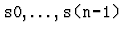into the range'' sort sn (an exception to this rule about intended meanings of sorts is discussed in the Chapter 7). A language distinguishes certain sorts as types and assigns a type to every sort. Distinct types are intended to denote sets of elements which need not be related. prop is a base'' type which belongs to every language and which is intended to denote the set of truth values. The sorts for h-o-real-arithmetic are given in Table 3.2. Table 3.2: Sorts for h-o-real-arithmetic  Sort Type of Sort Expressions of given sort nn ind iota(k:nn,k^2=9) zz ind [-1], 0, 1 qq ind [3/2] rr ind iota(x:rr,x^2=2) ind ind with(x:ind,x) prop prop truth, falsehood The expressions of a language are built from language constants and variables as specified by Table 3.3 below. A constant is a character string specified as such by the def-language form. A variable is also character string. Variables however, must begin with a text character (that is, an alphabetic character or one of the symbols _ %$ &) and contain only text characters or digits. Moreover, the sorting of a variable within an expression must be specified either by an enclosing binder or by an enclosing with'' declaration.

Table 3.3: String Syntax for Constructors
 Expression Category Syntax Sort Truth truth prop Falsehood falsehood prop Negation not(p) prop Conjunction p1 and ... and pn prop Disjunction p1 or ... or pn prop Implication p1 implies p2 prop Biconditional p1 iff p2 prop If-then-else formula if_form(p1,p2,p3) prop Universal forall(v1:s1,...,vn:sn,p) prop Existential forsome(v1:s1,...,vn:sn,p) prop Equality t1=t2 prop Application f(t1,...,tn) r Abstraction lambda(v1:s1,...,vn:sn,t) [s1,...,sn,s] Definite description iota(v:s,p) s If-then-else term if(p,t1,t2) s1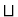s2 Definedness #(t) prop Sort-definedness #(t,s) prop Undefined expression ?s s With with(v1:s1,...,vn:sn,t) s
Notes:
1.
p, p1 ...pn are syntactic variables which denote formulas, that is, expressions of sort prop. t, t1 ...tn denote arbitrary expressions of sort s, s1 ...sn.
2.
s1s2 is the smallest sort which syntactically includes s1 and s2.
3.
For equality and if-then-else term, t1, t2 must be of the same type.
4.
For application, f is an arbitrary expression of sort [r1,...,rn,r], and the sorts ri and si must have the same enclosing type.
5.
With is not a constructor; it is merely a device to specify the sorts of free variables.

In the theory h-o-real-arithmetic there is additional syntax for arithmetic operators, described in Table 3.4. For example, - is used for both sign negation and the binary subtraction operator. The binary operators are all infix with different binding powers to reduce parentheses on input and output: In order of increasing binding power, the arithmetic operators are + * / ^ -.

Table 3.4: Syntax for h-o-real-arithmetic
 Term Category Syntax Sort Sum t1+ ... +tn rr Product t1* ... *tn rr Sign negation -t rr Subtraction t1-t2 rr Exponentiation t1^t2 rr Division t1/t2 rr Sequential sum sum(t1,t2,t3) rr Sequential product prod(t1,t2,t3) rr Factorial t! rr Less than t1t2 prop Greater than or equals t1>=t2 prop
Notes:
1.
The terms t1 ...tn must be of type ind. Do not confuse this syntactic requirement for well-formedness with semantic conditions for well-definedness.
2.
For sequential sum and product, the term t3 must be of type [ind,ind].
3.
All operators associate on the left except exponentiation which associates on the right.

To check the syntax of an expression (enclosed in double quotes), select the entry Check expression syntax in the pane IMPS help. IMPS will request an expression, which you can supply by clicking the right mouse button on the formula. You can also type the formula directly in the minibuffer. As an exercise, build the formula which asserts that for every nonnegative integer n the sum of squares of the first n integers is n (n+1) (2 n+1)/6.

# 3.5 Proofs

A formula in a theory is valid if it is true in every legitimate'' model of the theory; if the formula has free variables, we have to add: with every legitimate assignment of values to its free variables.'' To prove a formula means to offer conclusive mathematical evidence of its validity. The rules for admissible evidence are given by something called a proof system. In this section we will describe the IMPS proof system.

To begin an interactive proof in IMPS, select the start dg option in the Deduction Graphs pane of the menu. For version 19, click on the menubar on DG and then select Start dg. IMPS will then prompt you in the minibuffer with the text

   Formula or reference number:

This is an IMPS request for some formula to prove. You can supply this formula by typing its reference number in the minibuffer. Alternatively, you can click the right mouse button on the formula (provided that it is enclosed between double quotes), and then press <RET>. You are then ready to begin proving the formula.

After telling Emacs to start the deduction, two new buffers will be created. One buffer, labelled starDeduction-graph-1* displays a graphical representation of a data structure called a deduction graph. The other Emacs buffer labeled starSequent-nodes-1* displays a textual representation of a data structure called a sequent node. Notice that the mode-line for the sequent node buffer also contains the text


h-o-real-arithmetic: Sqn 1 of 1.

This gives you the following information:
1.
h-o-real-arithmetic is the theory in which the proof is being carried out.
2.
You are currently looking at the first sequent node of the deduction graph.
3.
There is only one sequent node in the deduction graph.

To understand the significance of the new buffer, note that a deduction graph provides a snapshot of the current status of the proof. A deduction graph is a directed graph with nodes of two kinds, called sequent nodes and inference nodes. An interactive proof in IMPS is carried out by adding inference nodes and sequent nodes to the deduction graph.

A sequent node consists of the following:

• A theory.
• A single formula B called the sequent node assertion.
• A set of formulas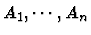called the sequent node assumptions.
Logically, a sequent node just represents the formula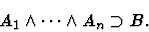In the starSequent-nodes-1* buffer, a sequent node is represented as a list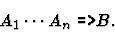Inference nodes are to be understood in part by their relationship to sequent nodes: Every inference node i has a unique conclusion node c and a (possibly empty) set of hypothesis nodes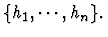An inference node represents the following mathematical relationship between c and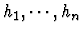: if every one of the sequent nodesis valid, then the sequent node c is valid. Alternatively, we can view this as asserting that the validity of the sequent node c can be reduced to the validity of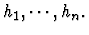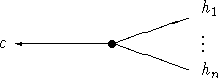An inference node also contains other information, providing the mathematical justification for asserting the relationship between candThis justification is called an inference rule.

Note that if the inference node i has no hypotheses, then c is obviously valid. When this is the case, c is said to be immediately grounded. More generally, c is valid if all the hypothesesof i are valid. Thus validity propagates from hypotheses to conclusion. A sequent node which is recognized as valid in this way is said to be grounded. The system indicates that it has recognized validity of a sequent node when it marks it as grounded. A proof is complete when the original sequent node of the deduction graph (i.e., the deduction graph's goal node) is marked as grounded.

Sequent nodes and inference nodes are added to a deduction graph using deduction graph commands. Some commands correspond to the use of one inference rule; for example extensionality or force-substitution fall into this category. Applying such a command either does nothing or adds exactly one inference node to the deduction graph. Moreover, this one inference node corresponds quite closely to the application of one mathematical fact. Other commands such as simplify have similar behavior in that they either do nothing or add exactly one inference node. Yet they are fundamentally different because behind the scene they are often carrying out thousands of very low-level inferences, such as logical and algebraic simplification and rewrite-rule application. Finally a third class of commands are essentially strategies developed to systematically apply primitive commands in search of a proof.

# 3.6 Extending IMPS

IMPS can be extended in the following ways:

• By adding definitions to an existing theory.
• By building a new theory altogether, with new primitive constants, sorts, and axioms.
For example, in the theory of h-o-real-arithmetic, evaluation of the following s-expression adds the definition of convergence to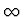of a real-valued sequence:
   (def-constant CONVERGENT%TO%INFINITY
"lambda(s:[zz,rr],
forall(m:rr, forsome(x:zz, forall(j:zz,
x<=j implies m<=s(j)))))"
(theory h-o-real-arithmetic))

As an exercise, try defining the concept of limit of real-valued sequences. Next, we illustrate how new theories are built by building a theory of monoids. Building a theory is a two-step process. First, we build a language with the required sorts and constants:
   (def-language MONOID-LANGUAGE
(embedded-languages h-o-real-arithmetic)
(base-types uu)
(constants
(e "uu")
(** "[uu,uu,uu]")))

The next step builds the theory itself by listing the axioms:
   (def-theory MONOID-THEORY
(component-theories h-o-real-arithmetic)
(language monoid-language)
(axioms
(associative-law-for-multiplication-for-monoids
"forall(z,y,x:uu, x**(y**z)=(x**y)**z)" rewrite)
(right-multiplicative-identity-for-monoids
"forall(x:uu,x**e=x)" rewrite)
(left-multiplicative-identity-for-monoids
"forall(x:uu,e**x=x)" rewrite)
("total_q(**,[uu,uu,uu])" d-r-convergence)))


There are numerous other ways IMPS can be extended. These are discussed in detail in Chapter 17. In addition, there are a number of annotated files which show you how theories are built and theorems proved in them. These are described in the chapters of this manual (and 5) on the micro exercises and the exercises.

# 3.7 The Little Theories Style

The IMPS methodology for formalizing mathematics is based on a particular version of the axiomatic method. The axiomatic method is commonly used both for encoding existing mathematics and for creating new mathematics. A chunk of mathematics is represented as an axiomatic theory consisting of a formal language plus a set of sentences in the language called axioms. The axioms specify the mathematical objects to be studied, and facts about the objects are obtained by reasoning logically from the axioms, that is, by proving theorems.

The axiomatic method comes in two styles, both well established in modern mathematical practice. We refer to them as the big theory'' version and the little theories'' version. In the big theory'' version all reasoning is performed within a single powerful and highly expressive axiomatic theory, such as Zermelo-Fraenkel set theory. The basic idea is that the theory we work in is expressive enough so that any model of it contains all the objects that will be of interest to us, and powerful enough so that theorems about these objects can be proved entirely within this single theory.

In the little theories version, a number of theories are used in the course of developing a portion of mathematics. Different theorems are proved in different theories, depending on the amount and kind of mathematics that is required. Theories are logically linked together by translations called theory interpretations which serve as conduits to pass results from one theory to another. We argue in  that this way of organizing mathematics across a network of linked theories is advantageous for managing complex mathematics by means of abstraction and reuse.

IMPS supports both the big theory and little theories versions of the axiomatic method. However, the IMPS little theories machinery of multiple theories and theory interpretations offers the user a rich collection of formalization techniques (described in Chapter 9) that are not easy to imitate in a strict big theory approach.Next: 4. On-Line Help Up: 1. Introductory Material Previous: 2. Distribution